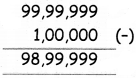Students can download 5th Maths Term 1 Chapter 2 Numbers Ex 2.4 Questions and Answers, Notes, Samacheer Kalvi 5th Maths Guide Pdf helps you to revise the complete Tamilnadu State Board New Syllabus, helps students complete homework assignments and to score high marks in board exams.

## Tamilnadu Samacheer Kalvi 5th Maths Solutions Term 1 Chapter 2 Numbers Ex 2.4

Question 1.
Write the number name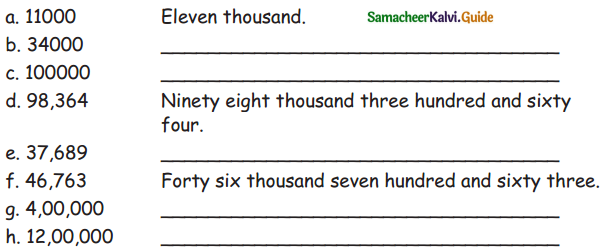a. 11000 – Eleven thousands
b. 34000 –  Thirty four thousands
c. 100000 – One lakh
d. 98,364 – Ninety eight thousand three hundred and sixty four
e. 37,689 – Thirty seven thousands six hundred and eighty nine
f. 46,763 – Forty six thousands seven hundred and sixty three
9- 4,00,000 – Four lakhs
h. 12,00,000 – Twelve lakhsQuestion 2.
Answer the following Represent given values in Abacus.

a. 3 tens crores, 7 crores, 6 ten lakhs, 7 lakhs, 4 tens and 7 ones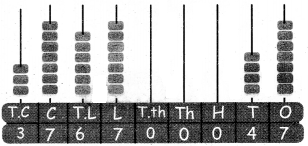3 tens crores, 7 crores, 6 ten lakhs, 7 lakhs, 4 tens and 7 ones = 37,67,00,047

b. Find the place value of 7 and 4 of this numbers 34578910.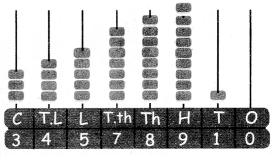The place value of 7 is 7 × 10000 = 70,000
The place value of 4 is 4 × 1000000 = 40,00,000

c. Write in numerals.
a. One crore forty thousands and four.
b. Sixty four lakhs and three.
a. One crore forty thousands and four.
= 1,00,40,004

b. Sixty four lakhs and three.
= 64,00,003d. Write the number names of the following numbers represented in the Abacus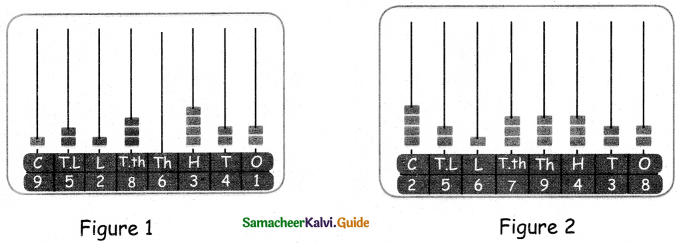Figure 1
9,52,86,341
Nine crores Fifty two lakhs eighty six thousands three hundred and forty one.

Figure 2
2,56,79,438
Two crores fifty six lakhs seventy nine thousands four hundred and thirty eight.

e. How many lakhs and hundreds are there in the numbers represented by the Abacus given above.
Ans:
Figure 1: There are 2 lakhs and 3 hundreds.
Figure 2: There are 6 lakhs and 4 hundreds.

f. Find the sum of greatest 4-digit number and smallest 5-digit numbers.
The greater 4 digit number = 9,999
The smallest 5 digit number = 10,000
Sum = 9,999 + 10,000
= 19,999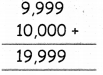g. Write the following ascending and descending order.
a. 33,058; 40,978; 97,879; 81,421; 90,470; 47,224
b. 99,999; 11,111; 22,222; 33,333; 44,444; 66,666
a. 33,058; 40,978; 97,879; 81,421; 90,470; 47,224
Ascending order: 33,058; 40,978; 47,224; 81,421; 90,470; 97,879
Descending order: 97,879; 90,470; 81,421; 47,224; 40,978; 33,058

b. 99,999; 11,111; 22,222; 33,333; 44,444; 66,666
Ascending order: 11,111; 22,222; 33,333; 44,444; 66,666; 99,999
Descending order: 99,999; 66,666; 44,444; 33,333; 22,222; 11,111h. Write in standard form: 7 lakhs + 5 thousands + 4 tens + 3 ones
7,05,043

i. Add 5 thousands and 3 hundreds to this number 1,34,510.